# Site map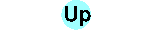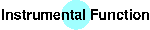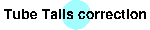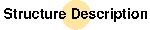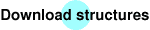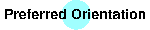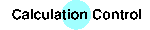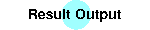In this step we want to build the necessary structure files for BGMN. The new `*.str` files can be prepared easily by using the program PowderCell, which is able to import several structure data files (e.g. ICSD, SHELX), to calculate the powder pattern and to save the structure in the `*.str` format.

The plaster consists mainly of three phases: quartz, calcite and gypsum. Lets have a look at the simple structure of quartz, which is provided in the free-format file `quartz.str`:

```PHASE=Quartz SpacegroupNo=154 //
PARAM=A=0.4913_0.49^0.4935 PARAM=C=0.5404_0.538^0.545 //
RP=3 PARAM=B1=0_0^0.01 GOAL=GrainSize(1,0,1) //
PARAM=GEWICHT=0_0 GOAL:quartz=GEWICHT
E=SI+4 Wyckoff=a TDS=0.002  x=0.47
E=O-2  Wyckoff=c TDS=0.0015 x=0.415 y=0.268 z=0.786
```
The explanation of the items is easy: You should provide a phase name and you must provide a space group number or a Hermann-Mauguin symbol. A list of all symbols is included in the file `spacegrp.dat`.

Then the lattice constants follow (`A`, `B`, `C`, `ALPHA`, `BETA`, `GAMMA`) with their starting values, lower (`_`) and upper (`^`) limits. The text `PARAM=` means that the following value is to be refined. All these parameters can have lower and/or upper limits. Lattice constants with fixed values of 90 or 120 deg or if `B=A` need no explicit entries in the structure file. They are automatically generated by the program based on the space group information.

The lattice constants and thermal displacement parameters are given in nm units. If one wants to use Å the entry `UNIT=ANGSTROEM` is necessary.

Nearly every phase has a size or microstrain broadening. With `RP=3 PARAM=B1=...` a Lorentzian crystallite size dependent broadening will be refined. The entry `GOAL=GrainSize...` calculates the crystallite size in the given lattice direction. If you want to refine additional microstrain broadening you have to write:

```RP=4 PARAM=B1=0_0^0.01 PARAM=k1=0_0^1 PARAM=k2=0_0
```
`k1` and `k2` are width parameters of a Gaussian-like broadening function. `k1` is a measure of the width of the size distribution and `k2` is the mean squared strain.

The weighting factor `GEWICHT` determines the share of the phase in the sample. It is comparable with the Rietveld scale factor, but it includes the X-ray density. Thats why the mass proportions of the phases later can easily be calculated from this parameter. Therefore the additional entry `GOAL:quartz=GEWICHT` is needed.

For elderly BGMN versions, the first three lines must have a `//` double-letter at the end to tell the program that no atomic positions follow.

Starting with level 3.3.1, this `//` delimiters are fully optional and may be written at the end of every line (at the end of the atomic position lines, too). They may be followed by any comment. Therefore, you may write the above example as follows:

```PHASE=Quartz SpacegroupNo=154
PARAM=A=0.4913_0.49^0.4935 PARAM=C=0.5404_0.538^0.545
RP=3 PARAM=B1=0_0^0.01 GOAL=GrainSize(1,0,1)
PARAM=GEWICHT=0_0 GOAL:quartz=GEWICHT
E=SI+4 Wyckoff=a TDS=0.002  x=0.47 // Silicon position
// follows oxygen position
E=O-2  Wyckoff=c TDS=0.0015 x=0.415 y=0.268 z=0.786
```

With `E=` atomic positions are defined. Elements or Ions have to be written in capital letters. A list of the available symbols is found in the file `afaparm.dat`.

A Wyckoff position is needed from which the program determines the free coordinates. These coordinates must be provided as constants as in the example or as refineable parameters with the `PARAM=` prefix. `TDS` is the isotropic thermal displacement value for the atomic position (in nm^2). It can also be a refineable parameter.

An additional explanation of the variables possible is available.